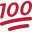# Android Code Snippet[B4X] Validate and verify CIF, NIF, DNI and Others

Validate and verify NIF, CIF and DNI and can be expanded to other verifiers

attached demo

B4X:
``````Private Sub Button1_Click
Log(Cif_Validation("A58818501"))
Log(Cif_Validation("A12345678"))
Log(Cif_Validation("K5881850A"))
Log(Cif_Validation("12345678Z"))
End Sub

Public Sub Cif_Validation(CIF As String) As Boolean
Dim Result As Boolean = False
Select True
Case Regex.IsMatch("(^[XYZ\d]\d{7})([TRWAGMYFPDXBNJZSQVHLCKE]\$)", CIF.ToUpperCase)
Dim Matcher1 As Matcher = Regex.Matcher("(^[XYZ\d]\d{7})([TRWAGMYFPDXBNJZSQVHLCKE]\$)", CIF.ToUpperCase)
Matcher1.Find
Dim Control = "TRWAGMYFPDXBNJZSQVHLCKE" As String
Dim Checksum As Int = Matcher1.Group(1).Replace("X",0).Replace("Y",1).Replace("Z",2)
Dim Digit As String = Control.CharAt(Checksum Mod 23)
Result = Matcher1.Group(2) = Digit

Case Regex.IsMatch("(^[ABCDEFGHIJKLMUV])(\d{7})(\d\$)", CIF.ToUpperCase)
Dim Matcher1 As Matcher = Regex.Matcher("(^[ABCDEFGHIJKLMUV])(\d{7})(\d\$)", CIF.ToUpperCase)
Matcher1.Find
Dim Checksum = 0, Pos = 0 As Int
For Each Val As Int In Regex.Split("", Matcher1.Group(2))
Dim Sum As Int
For Each Num As Int In Regex.Split("", (Val*(2-(Pos Mod 2))).As(String))
Sum = Sum + Num
Next
Checksum = Checksum + Sum
Pos = Pos + 1
Next
Checksum = ((10 - (Checksum Mod 10)) Mod 10)
Result = Matcher1.Group(3) = Checksum

Case Regex.IsMatch("(^[KLMNPQRSW])(\d{7})([JABCDEFGHI]\$)", CIF.ToUpperCase)
Dim Matcher1 As Matcher = Regex.Matcher("(^[KLMNPQRSW])(\d{7})([JABCDEFGHI]\$)", CIF.ToUpperCase)
Matcher1.Find
Dim Control = "JABCDEFGHI" As String
Dim Checksum = 0, Pos = 0 As Int
For Each Val As Int In Regex.Split("", Matcher1.Group(2))
Dim Sum As Int
For Each Num As Int In Regex.Split("", (Val*(2-(Pos Mod 2))).As(String))
Sum = Sum + Num
Next
Checksum = Checksum + Sum
Pos = Pos + 1
Next
Dim Digit As String = Control.CharAt(((10 - (Checksum Mod 10)) Mod 10))
Result = Matcher1.Group(3) = Digit
End Select
Return Result
End Sub``````

#### Attachments

• CifAndNif.zip
3.2 KB · Views: 113
Last edited:
••grantorax, scsjc, jotaeme and 6 others